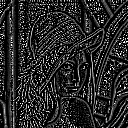## SHARP

 Adaptively sharpens an image or extract edges.

 USAGE: sharp [-m method] [-s sharpen] infile outfile USAGE: sharp [-st] infile USAGE: sharp [-h or -help] -m ............ method ....... method=0 is edge extract; method=1 is sharpen .............................. default=1 -f ............ factor ....... sharpening/edge extraction gain factor; .............................. factor>=0; float; default=2 -st .......................... get image statistics -h or -help .................. get help PURPOSE: To sharpen an image or extract edges adaptively. DESCRIPTION: SHARP is an adaptive technique to either sharpen an image or extract edges. It applies an adaptive gain factor based upon the image's local standard deviation to a high pass filtered version of the image and adds that to a low pass filtered version of the image. The adaptive formula R = method*M + G*H. Here R is the resulting image. M is the low pass filtered image which is the local mean image generated by applying a 3x3 average convolution to the input image, I. H = (I - M) is the high pass filtered image. G is the gain image, which is computed as G = (factor*std)/(S + (factor*std/mg)). Here std is the image's global standard deviation, mg is a maximum gain constant and S is the image's local standard deviation in the 3x3 neighborhood. ARGUMENTS: -m method - method is either 0 or 1. A value of 0 for method indicates edge extraction and value of 1 for method indicates sharpening. The default=1. -f factor factor is the sharpening/edge extraction gain factor. It is a multiplier to the image's actual standard deviation. The value for factor must be greater than or equal to 0. A value of about 0.5 leaves the image nearly unchanged. A smaller value blurs the image or extract more edges. A larger value sharpens the image or extracts fewer edges. This transition value is not exact and is likely image statistics dependent as the result is a mix of the low pass filtered image (not the original image) and the adaptive high pass filtered image. Factor is floating point number. The default=2. CAVEAT: No guarantee that this script will work on all platforms, nor that trapping of inconsistent parameters is complete and foolproof. Use At Your Own Risk.

### EXAMPLES

 Adaptive Sharpening/Blurring Of A True Color Image Original Image Arguments: -m 1 -f 0 (blurring) Arguments: -m 1 -f 1.2 (sharpening) Arguments: -m 1 -f 2 (sharpening) Arguments: -m 1 -f 10 (sharpening)Adaptive Edge Extraction From A Grayscale Image Original Image Arguments: -m 0 -f 0 Arguments: -m 0 -f 0.5 Arguments: -m 0 -f 2 Arguments: -m 0 -f 10What the script does is as follows: Computes a local mean image using a uniform (simple average) convolution. Computes a local standard deviation image using the input image and the local mean image. Computes a gain image using the local standard deviation image and the global standard deviation of the image. Combines the local mean image and the product of the local gain image times the difference between the input image and the local mean image. This is equivalent to the following IM commands for the case of adaptive sharpening (-m 1 -f 2): ave="1,1,1,1,1,1,1,1,1" convert \$infile -convolve "\$ave" \$tmpM convert \( \$infile \$infile -compose multiply -composite -convolve "\$ave" \) \ \( \$tmpM \$tmpM -compose multiply -composite \) +swap \ -compose minus -composite -fx "sqrt(u)" \$tmpS method=1 factor=2 std=`echo "\$data" | sed -n '/^.*Standard.*[(]\([0-9.]*\).*\$/{ s//\1/; p; q; }'` std=`echo "scale=1; \$std * 100 / 1" | bc` dstd=`echo "scale=5; \$factor * \$std / 100" | bc` dsdmg=`echo "scale=5; \$dstd / \$mg" | bc` gain="gn=(\$dstd)/(u+(\$dsdmg));" convert \$infile \$tmpM \$tmpS -fx "\$gain ((\$method*v)+(gn*(u-v)))" \$outfile